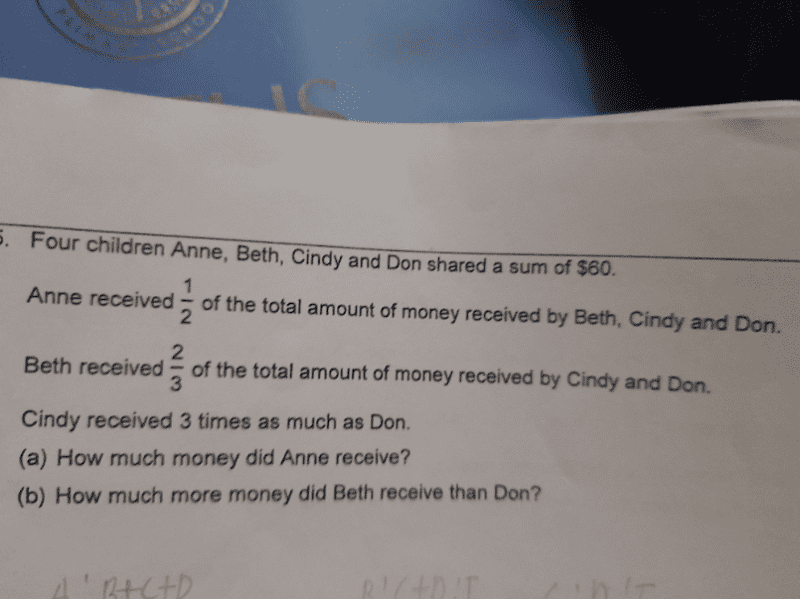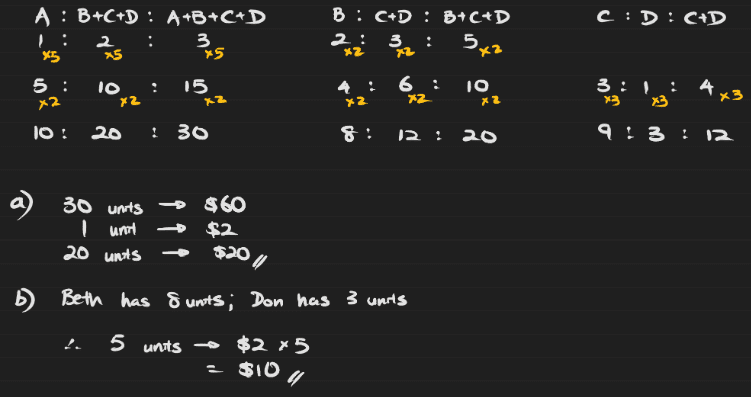# Question0 Replies 1 Like

(1) A+B+C+D = 60

(2) B+C+D = 2A

(3) C+D = (3/2)B

(4) D = (1/3)C

a) Substituting (2) into (1):
3A = 60
So A = \$20 #

b) Substituting (3) into (2):
(5/2)B = 2A
But A=20, so B = (2/5)(40) = \$16

Substituting (4) into (3):
(4/3)C = (3/2)B
But B=16, so C = (3/4)(24) = \$18

So D = (1/3)(18) = \$6

Therefore B received \$16-\$6 = \$10 more than D.

I did this by simultaneous equations… would be grateful if someone can come up with the model answer.

0 Replies 1 Like